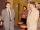Container

First day got off 1/4 water from full container, second day 1/5 of the remaining water. How many percent remain container filled?

Result

p =  60 %

Solution:

1 - 1/4 - 1/5*(1-1/4) = p/100

p = 60

p = 60

Calculated by our simple equation calculator.

Leave us a comment of this math problem and its solution (i.e. if it is still somewhat unclear...):Be the first to comment!To solve this verbal math problem are needed these knowledge from mathematics:

Our percentage calculator will help you quickly calculate various typical tasks with percentages. Do you have a linear equation or system of equations and looking for its solution? Or do you have quadratic equation?

Next similar math problems:

1. Tickets1260 tickets sold. On the first day, 80% was sold on the second day was sold. How many tickets were sold first and how much the next day?
2. Two workersTwo workers make 138 parts/items. The first one produces 30% more than the second. How many items will each produce?
3. Two numbersWe have two numbers. Their sum is 140. One-fifth of the first number is equal to half the second number. Determine those unknown numbers.
4. The percentages in practiceIf every tenth apple on the tree is rotten it can be expressed by percentages: 10% of the apples on the tree is rotten. Tell percent using the following information: a. in June rained 6 days b, increase worker pay 500 euros to 50 euros c, grabbed 21 fro
5. TVsProduction of television sets increased from 3,500 units to 4,200 units. Calculate the percentage of production increase.
6. The ballThe ball was discounted by 10 percent and then again by 30 percent. How many percent of the original price is now?
7. Apples 2James has 13 apples. He has 30 percent more apples than Sam. How many apples has Sam?
8. Waiting roomIn the waiting room are people and flies. Together they have 15 heads and 50 legs (fly has 6 legs). How many people and flies are in the waiting room?
9. The dormitoryThe dormitory accommodates 150 pupils in 42 rooms, some of which are triple and some are quadruple. Determine how many rooms are triple and how many quadruples.
10. Boys and girlsThere are 48 children in the sports club, boys are 10 more than girls. How many girls go to the club?
11. New refrigeratorNew refrigerator sells for 1024 USD, Monday will be 25% discount. How much USD will save, and what will be the price?
12. EnrollmentThe enrollment at a local college increased 4% over last year's enrollment of 8548. Find the increase in enrollment (x1) and the current enrollment (x2).
13. MeatHalf a kilogram of pork and three-quarters of a kilogram of beef cost total 5.1 USD. One quarter of a kilogram of beef and 1 kilogram of pork cost 4.9 USD. Determine the price of 1 kg of each type of meat.
14. Linear systemSolve this linear system (two linear equations with two unknowns): x+y =36 19x+22y=720
15. Substitutionsolve equations by substitution: x+y= 11 y=5x-25
16. Belgium vs ItalyBelgium played a match with Italy and Belgium win by 2 goals. The match fell a total 6 goals. Determine the number of goals scored by Belgium and by Italy.
17. Fifth of the numberThe fifth of the number is by 24 less than that number. What is the number?